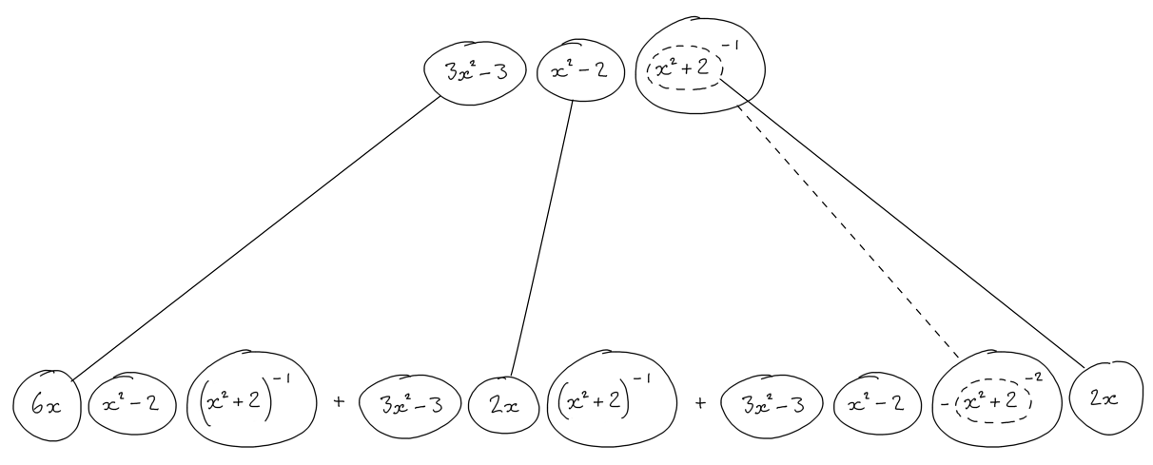# Thread: How do I find the derivative of this problem?

1. ## How do I find the derivative of this problem?

f(x)=x2+2(3x2−3)(x2−2)Do I just multply out the top to get f(x) and then get the derivative by the quotient rule from there?

I got what I thought was the derivative: (12x^2(X^2+2)-(3x^2-3)(x^2-2)(2x))/(x^2+2)^2 but it said I was incorrect.

2. ## Re: How do I find the derivative of this problem?Originally Posted by rhcprule3f(x)=x2+2(3x2−3)(x2−2)Do I just multply out the top to get f(x) and then get the derivative by the quotient rule from there?
There is no top or bottom in what you have written; the whole formula is on one line.

In plain text, it is customary to write x^2 for x².

3. ## Re: How do I find the derivative of this problem?

You seem to have forgotten a "/"! Did you mean (x^2+ 2)(3x^2- 3)/(x^2- 2)?
(You also seem to have forgotten the parentheses around x^2+ 2. Or did you really mean "x^2+ [2(3x^2- 3)]= x^2+ 6x^2- 6= 7x^2- 6 as you wrote?)

By the quotient rule, the derivative is [((x^2+2)(3x^2- 3))'(x^2- 2)- (x^2+2)(3x^2-3)(x^2- 2)']/(x^2-2)^2.
You can do the derivative of the numertor, ((x^2+2)(3x^2- 3))' either by first multiplying it out or by using the product rule.

4. ## Re: How do I find the derivative of this problem?

sorry the problem was supposed to be (3x^2-3)(x^2-2) all over (x^2+2)

5. ## Re: How do I find the derivative of this problem?Originally Posted by rhcprule3sorry the problem was supposed to be (3x^2-3)(x^2-2) all over (x^2+2)
Use logarithmic differentiation: $\displaystyle y' = \frac{{(3{x^2} - 3)({x^2} - 2)}}{{\left( {{x^2} + 2} \right)}}\left[ {\frac{{6x}}{{(3{x^2} - 3)}} + \frac{{2x}}{{({x^2} - 2)}} - \frac{{2x}}{{({x^2} + 2)}}} \right]$

6. ## Re: How do I find the derivative of this problem?Originally Posted by rhcprule3sorry the problem was supposed to be (3x^2-3)(x^2-2) all over (x^2+2)
y = (3x^2-3)(x^2-2)/(x^2+2)
This is of the form y = u/v ==> (vu' - v'u)/v^2

Can you continue from there?

7. ## Re: How do I find the derivative of this problem?

Plato's point being that if $\displaystyle y= \frac{(3x^2-3)(x^2- 2)}{x^2+ 2}$ then $\displaystyle ln(y)= ln\left(\frac{(3x^2-3)(x^2- 2)}{x^2+ 2}\right)= ln(3x^2- 3)+ ln(x^2- 2)- ln(x^2+ 2)$ so that $\displaystyle d(ln(y))/dx= (1/y)(dy/dx)= 6x/(3x^2- 3)+ 2x/(x^2- 2)- 2x/(x^2+ 2)$ and then
$\displaystyle dy/dx= [(3x^2- 3)(x^2- 2)/(x^2+ 2)]6x/(3x^2- 3)+ 2x/(x^2- 2)- 2x/(x^2+ 2)$

8. ## Re: How do I find the derivative of this problem?

I've tried using the quotient rule to get the derivative but the webwork still says im incorrect

9. ## Re: How do I find the derivative of this problem?Originally Posted by rhcprule3I've tried using the quotient rule to get the derivative but the webwork still says im incorrect
Show us your work we will help you finding what you missed

10. ## Re: How do I find the derivative of this problem?Originally Posted by rhcprule3I got what I thought was the derivative: (12x^2(X^2+2)-(3x^2-3)(x^2-2)(2x))/(x^2+2)^2 but it said I was incorrect.Originally Posted by rhcprule3I've tried using the quotient rule to get the derivative but the webwork still says im incorrect
You have now been shown many different ways of finding the solution. If you got the answer wrong before, then try the quotient rule again. You did it wrong when you tried it before.

Break it down. You have $\displaystyle f(x) = \dfrac{u(x)}{v(x)}$. Write out the functions $\displaystyle u(x)$ and $\displaystyle v(x)$. Then find the derivatives $\displaystyle u'(x)$ and $\displaystyle v'(x)$. Write them here for us to check, and we can figure out where you might be going wrong. From your answer in your first post, it appears you think the derivative of $\displaystyle (3x^2-3)(x^2-2)$ is $\displaystyle 12x^2$. Is that the case?

11. ## Re: How do I find the derivative of this problem?

yes i believe the derivative is 12x^2 from u(x) and I belive the derivative for v(x) is 2x

12. ## Re: How do I find the derivative of this problem?

Then that is your problem. If $\displaystyle u(x) = (3x^2-3)(x^2-2)$, then $\displaystyle u'(x) \neq 12x^2$. Use the product rule (as HallsofIvy said).

13. ## Re: How do I find the derivative of this problem?

Ive tried everything I got u'(x) to equal 12x^3-18x. So I did the quotient rule: (36x^2-18)(x^2+2)-2x(12x^3-18x) all over (x^2+2)^2. I've tried foiling and product rule on (36x^2-18)(x^2+2) but I still ended up with the wrong anser and its EXTREMELY frustrating!!!

14. ## Re: How do I find the derivative of this problem?

You'll feel better when you realise that you've made errors, which is more reassuring than if the maths weren't capable of behaving itself! So re-read the given solutions. Here's another:... where (key in spoiler) ...

Spoiler:... is the chain rule. Straight continuous lines differentiate downwards (integrate up) with respect to the main variable (in this case x), and the straight dashed line similarly but with respect to the dashed balloon expression (the inner function of the composite which is subject to the chain rule).

But this is wrapped inside the legs-uncrossed version of a triple version of...... the product rule, where, again, straight continuous lines are differentiating downwards with respect to x.

Full size

__________________________________________________ __________

Don't integrate - balloontegrate!

Balloon Calculus; standard integrals, derivatives and methods

Balloon Calculus Drawing with LaTeX and Asymptote!

15. ## Re: How do I find the derivative of this problem?

Ive tried for f'(2) by plugging 2 for the x values but its still not giving it to me.... even with the product rule youve told me to use...

#### Search Tags

derivative, problem